# Electrical and Electronic Measurements - Online Test

Q1.
The bridge circuit shown in the figure below is used for the measurement of an unknown element ZX. The bridge circuit is best suited when ZX is a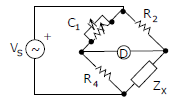Explaination / Solution:

Q2. Consider the following statement (i) The compensating coil of a low power factor wattmeter compensates the effect of the impedance of the current coil. (ii) The compensating coil of a low power factor wattmeter compensates the effect 0of the impedance of the voltage coil circuit.
Explaination / Solution:
No Explaination.

Q3.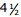digit DMM has the error specification as : 0.2% of reading + 10 counts. If a dc voltage of 100V is read on its 200V full scale, the maximum error that can be expected in the reading is
Explaination / Solution:
No Explaination.

Q4. The bridge method commonly used for finding mutual inductance is
Explaination / Solution:
No Explaination.

Q5. In the circuit shown below, the current through the inductor isExplaination / Solution:Q6. For the circuit shown in the figure, the voltage and current expressions are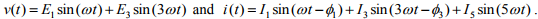The average power measured by the Wattmeter is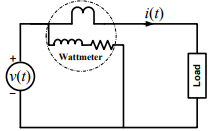Explaination / Solution: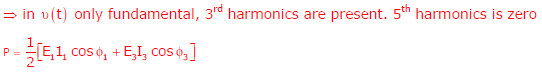Q7. An analog voltmeter uses external multiplier settings. With a multiplier setting of 20 kΩ it reads 440 V and with a multiplier setting of 80 kΩ it reads 352 V. For a multiplier setting of 40 kΩ the voltmeter reads
Explaination / Solution: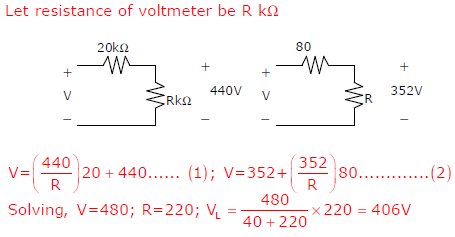Q8. Three moving iron type voltmeters are connected as shown below. Voltmeter readings are V, V1 and V2 as indicated. The correct relation among the voltmeter readings is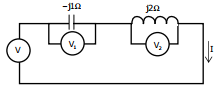Explaination / Solution:
No Explaination.

Q9.
The input impedance of the permanent magnet moving coil (PMMC) voltmeter is infinite. Assuming that the diode shown in the figure below is ideal, the reading of the voltmeter in Volts isExplaination / Solution:
No Explaination.

Q10. A strain gauge forms one arm of the bridge shown in the figure below and has a nominal resistance without any load as Rs = 300 Ω. Other bridge resistances are R1 = R2 = R3 = 300 Ω. The maximum permissible current through the strain gauge is 20 mA. During certain measurement when the bridge is excited by maximum permissible voltage and the strain gauge resistance is increased by 1% over the nominal value, the output voltage V0 in mV is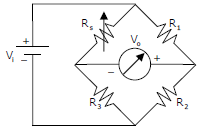Explaination / Solution:
No Explaination.